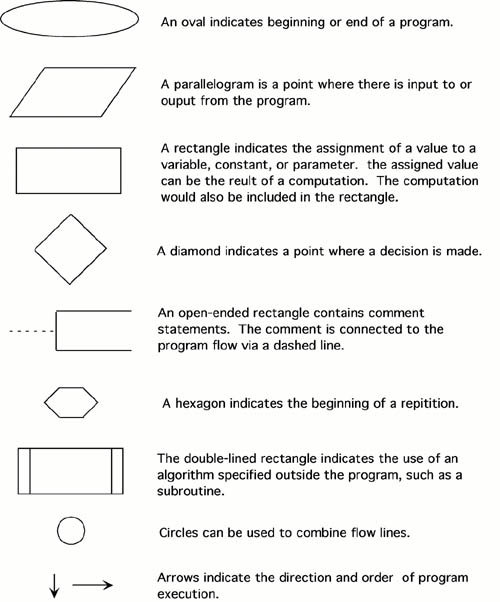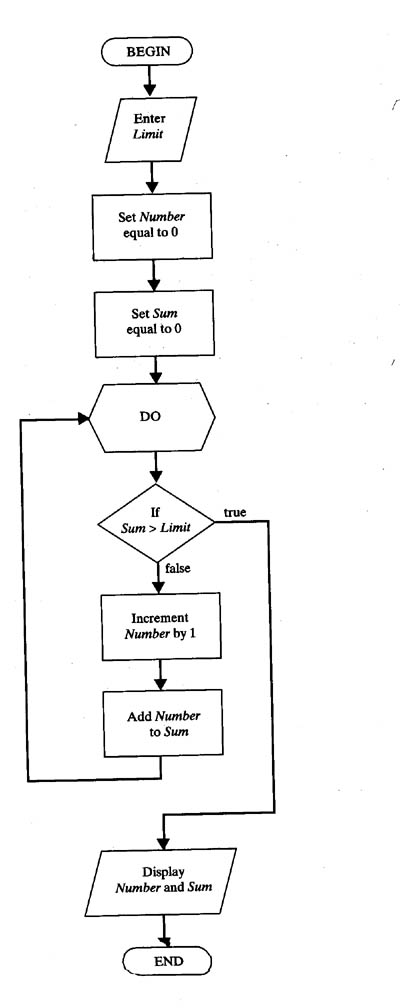« previous next»

3.3    Pseudocode and Flowcharts

Good, logical programming is developed through good pre-code planning and organization.  This is assisted by the use of pseudocode and program flowcharts.

Flowcharts are written with program flow from the top of a page to the bottom.  Each command is placed in a box of the appropriate shape, and arrows are used to direct program flow.  The following  shapes are often used in flowcharts:Pseudocode is a method of describing computer algorithms using a combination of natural language and programming language.  It is essentially an intermittent step towards the development of the actual code.  It allows the programmer to formulate their thoughts on the organization and sequence of a computer algorithm without the need for actually following the exact coding syntax.  Although pseudocode is frequently used there are no set of rules for its exact implementation.  In general, here are some rules that are frequently followed when writing pseudocode:

• The usual Fortran symobols are used for arithmetic operations (+, -, *, / , **).
• Symbolic names are used to indicate the quantities being processed.
• Certain Fortran keywords can be used, such as PRINT, WRITE, READ, etc.
• Indentation should be used to indicate branches and loops of instruction.

Here is an example problem, including a flowchart, pseudocode, and the final Fortran 90 program.  This problem and solution are from Nyhoff, pg 206:

For a given value, Limit, what is the smallest positive integer Number for which the sum

Sum = 1 + 2 + ... + Number

is greater than Limit.  What is the value for this Sum?

Pseudocode:

Input:    An integer Limit
Ouput:   Two integers: Number and Sum

1.  Enter Limit
2.  Set Number = 0.
3.  Set Sum = 0.
4.  Repeat the following:
a.  If Sum > Limit, terminate the repitition, otherwise.
b.  Increment Number by one.
c.  Add Number to Sum and set equal to Sum.
5.  Print Number and Sum.

Flowchart:Fortran 90 code:

PROGRAM Summation

!  Program to find the smallest positive integer Number
!  For which Sum = 1 + 2 + ... + Number
!  is greater than a user input value Limit.

IMPLICIT NONE

!  Declare variable names and types

INTEGER :: Number, Sum, Limit

!  Initialize Sum and Number

Number = 0
Sum = 0

!  Ask the user to input Limit

PRINT *, "Enter the value for which the sum is to exceed:"

!  Create loop that repeats until the smallest value for Number is found.

DO
IF (Sum > Limit) EXIT    !  Terminate repetition once Number is found
!  otherwise increment number by one
Number = Number + 1
Sum = Sum + 1
END DO

!  Print the results

PRINT *, "1 + ... + ", Number, "=", Sum, ">", Limit

END PROGRAM

 « previous next»